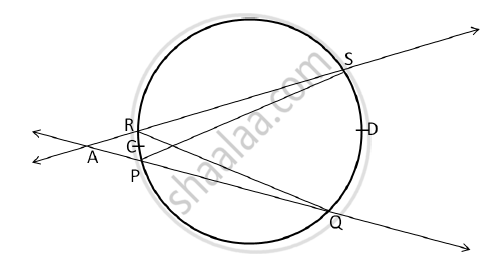# In the following figure, secants containing chords RS and PQ of a circle intersects each other in point A in the exterior of a circle if m(arc PCR) = 26°, m(arc QDS) = 48°, then find - Geometry

In the following figure, secants containing chords RS and PQ of a circle intersects each other in point A in the exterior of a circle if m(arc PCR) = 26°, m(arc QDS) = 48°, then find:
(i) m∠PQR
(ii) m∠SPQ
(iii) m∠RAQ#### Solution

Given: m(arc PCR) = 26°, m(arc QDS) = 48°
By Inscribed Angle Theorem,we get

(i) ∠PQR = 1/2 m(arc PCR)

∠PQR = 1/2 x 26º

∠PQR = ∠AQR = 13º ...(1)

(ii) ∠SPQ = 1/2m(arc QDS)

∠SPQ = 1/2 x  48º

∠SPQ = 24º ...(2)

(iii) In ΔAQR, by the Remote Interior Angle theorem,

∠RAQ + ∠AQR = ∠SRQ

∠SRQ = ∠SPQ ...(Angles subtended by the same arc)

i.e. ∠RAQ + ∠AQR = ∠SPQ

m ∠RAQ = m ∠SPQ - m∠AQR

m ∠RAQ = 24º - 13º ...[From (1) and (2)]

m ∠RAQ = 11º

Concept: Angle Subtended by the Arc to the Point on the Circle
Is there an error in this question or solution?